Get instant live expert help with Excel or Google Sheets“My Excelchat expert helped me in less than 20 minutes, saving me what would have been 5 hours of work!”

#### Post your problem and you’ll get expert help in seconds.

Your message must be at least 40 characters
Our professional experts are available now. Your privacy is guaranteed.

# How to Create a Stratified Random Sample in Excel

Excel allows us to create a stratified random sample using the RAND, COUNTIFS, IF, ROUND and COUNTA functions. This step by step tutorial will assist all levels of Excel users to create a random sample of the population based on the groups share in the population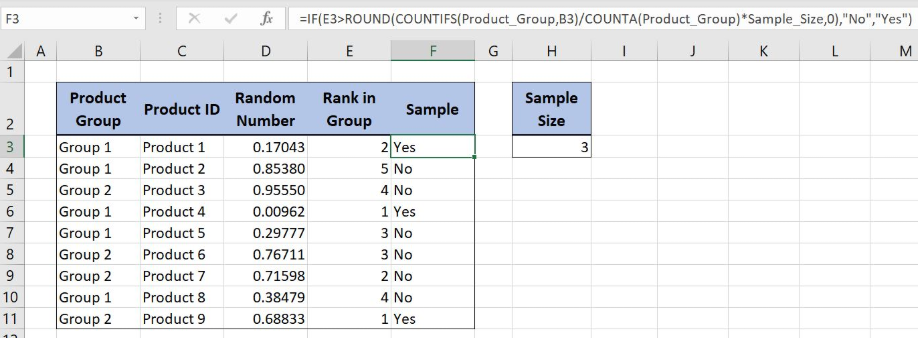Figure 1. Create a stratified random sample Excel

## Syntax of the RAND Formula

The generic formula for the RAND function is:

`=RAND()`

The function returns a random decimal number between 0 and 1 and has no parameters.

## Syntax of the COUNTIFS Formula

`=COUNTIFS(criteria_range1, criteria1, criteria_range2, criteria2, ... )`

The parameters of the COUNTIFS function are:

• criteria_range1, criteria_range2 – ranges where we want to apply our criteria
• criteria1, criteria2 – a criteria in criteria ranges which we want to count.

## Syntax of the IF Formula

`=IF(logical_test, value_if_true, value_if_false)`

The parameters of the IF function are:

• logical_test – a logical expression that we want to check
• value_if_true – a value which the function returns if a logical_test is TRUE
• value_if_false – a value which the function returns if a logical_test is FALSE.

## Syntax of the ROUND formula

`=ROUND(number, num_digits)`

The parameters of the ROUND function are:

• number – a decimal number which we want to round
• num_digits – a number of decimal places to which we want to round a number

## Syntax of the COUNTA Formula

`=COUNTA(range)`

The parameter of the COUNTA function is:

• range – a range of cells where we want to count non-blank cells.

## Setting up Our Data to Create a Stratified Random Sample in Excel

Our first table consists of 5 columns: “Product Group” (column B), “Product ID” (column C), “Random Number” (column D), “Rank in Group” (column E) and “Sample” (column F). The second table has one column “Sample Size” (column H). The idea is to create a stratified random sample based on the product groups, product ID and the sample size.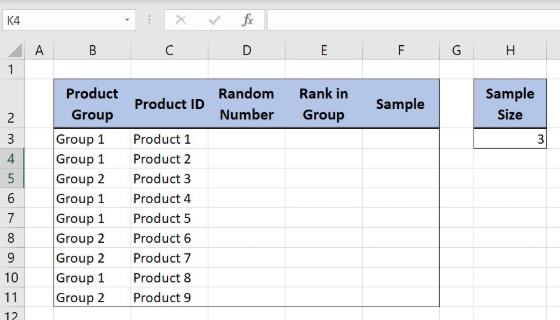Figure 2. Data for the example

## Create a Stratified Random Sample with RAND, COUNTIFS, IF, ROUND and COUNTA Functions

We want to create a stratified random sample of the data in column C based on the “Product Group” and “Sample Size”. The stratified random sampling is a way of creating the sample based on the groups share in the entire population. The population is divided into groups and the number of samples from each group is defined by group share in the entire population.

In order to make the formula more clear, we will create a named range Product_Group for cell range B3:B11, Random_Number for cell range D3:D11 and a named range Sample_Size for the cell H3.

To create a named range we should follow the steps:

• Select the cell range that should be named
• Click on the name box in Excel
• Write the name for the cell range and press enter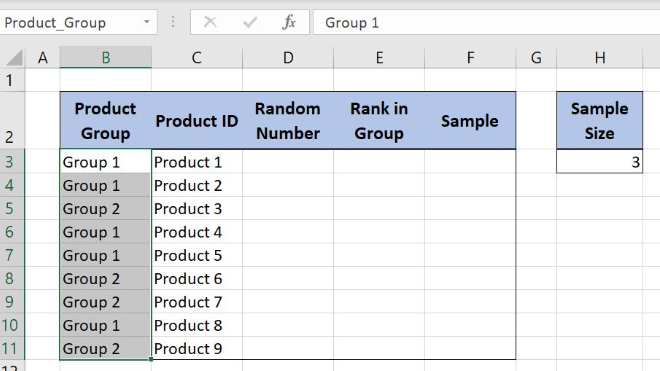Figure 3. Creating a named range Product_Group for column “Product Group”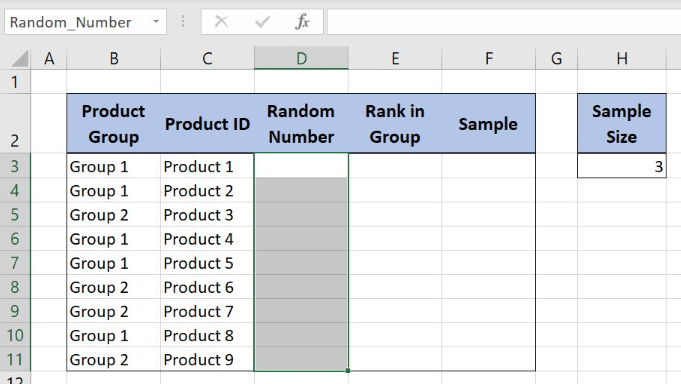Figure 4. Creating a named range Random_Number for column “Random Number”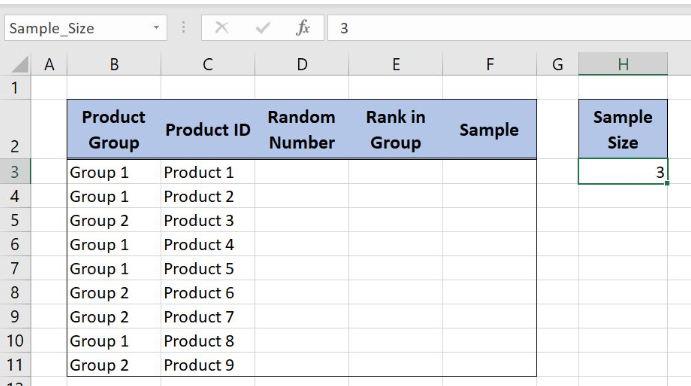Figure 5. Creating a named range Sample_Size for column “Sample Size”

he formula in Column D looks like:

`=RAND()`

The RAND function gets the random value from 0 to 1. The randomized values are placed in column D and will help us to get the stratified random sample in column F.

To apply the formula, we need to follow these steps:

• Select cell D3 and click on it
• Insert the formula: `=RAND()`
• Press enter.
• Drag the formula down to the other cells in the column by clicking and dragging the little “+” icon at the bottom-right of the cell.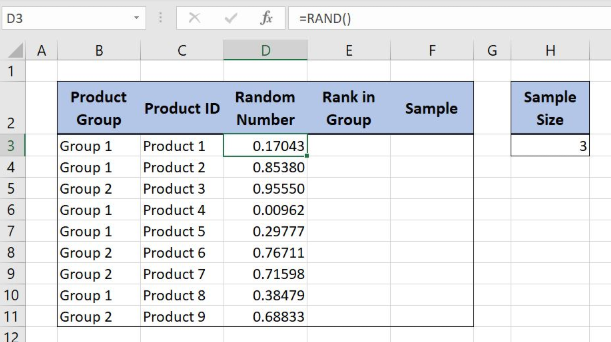Figure 6. Using the RAND formula to get a random value between 0 and 1

In column E we will place the formula to get the “Product ID” rank in each group:

`=1+COUNTIFS(Product_Group,B3,Random_Number,"<"&D3)`

The parameter criteria_range1 is a named range Product_Group while the criteria1 is the cell B3. The criteria_range2 is a named range Random_Number while the criteria2 are the cells that are less than the cell value D3, “<“&D3.

To apply the formula, we need to follow these steps:

• Select cell E3 and click on it
• Insert the formula: `=1+COUNTIFS(Product_Group,B3,Random_Number,"<"&D3)`
• Press enter
• Drag the formula down to the other cells in the column by clicking and dragging the little “+” icon at the bottom-right of the cell.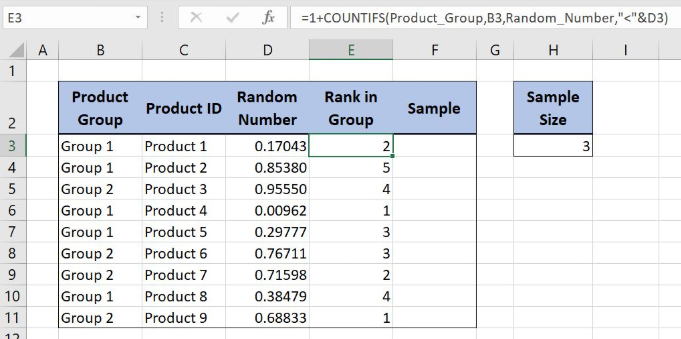Figure 7. Get the Product ID rank in each Product Group based on the Random Number

The first COUNTIFS criteria is counting the number of cells in each group. The other one is defining the Product ID rank in each group based on the Random Number from column D. The formula output is number 2 because the value in the cell D3 is the 2nd smallest value in column D for Group 1.

Now we can place the formula in column F to define the stratified random sample:

`=IF(E3>ROUND(COUNTIFS(Product_Group,B3)/COUNTA(Product_Group)*Sample_Size,0),"No","Yes")`

In IF function logical_test is the formula E3>ROUND(COUNTIFS(Product_Group,B3)/COUNTA(Product_Group)*Sample_Size,0) while the parameters value_if_true and value_if_false are the strings “No” and “Yes”. The ROUND function parameter number is the formula COUNTIFS(Product_Group,B3)/COUNTA(Product_Group)*Sample_Size while the num_digits parameter is 0.

The COUNTIFS function parameter criteria_range1 is a named range Product_Group while the criteria1 is the cell B3. Finally, the COUNTA function parameter range is a named range Product_Group.

To apply the formula, we need to follow these steps:

• Select cell F3 and click on it
• Insert the formula: `=IF(E3>ROUND(COUNTIFS(Product_Group,B3)/COUNTA(Product_Group)*Sample_Size,0),"No","Yes")`
• Press enter
• Drag the formula down to the other cells in the column by clicking and dragging the little “+” icon at the bottom-right of the cell.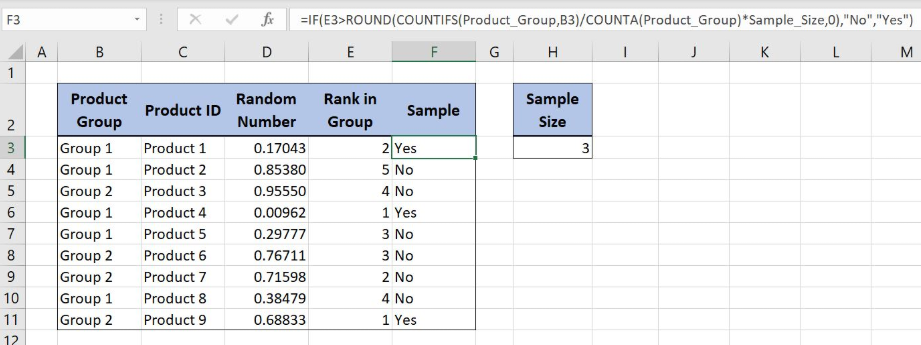Figure 8. Define a stratified random sample with IF, ROUND, COUNTIFS and COUNTA functions

The COUNTIFS function result is number 5 because there are 5 “Group 1” cells in column B. This number is divided with number 9, the total number of the non-blank cells in column B. Finally the result is multiplied with number 3 (sample size) to get the number of samples from the Group 1: ROUND(5/9*3,0). The ROUND function is converting the decimal number to an integer: ROUND (1,66666667,0) giving the result 2.

The IF function is checking if the rank value in the cell E3 is greater than the ROUND formula output: IF(2>2,”No”,”Yes”). The logical test value is FALSE and the final formula result is the string “Yes” indicating that Product 1 in Group 1 is included in the sample.

Most of the time, the problem you will need to solve will be more complex than a simple application of a formula or function. If you want to save hours of research and frustration, try our live Excelchat service! Our Excel Experts are available 24/7 to answer any Excel question you may have. We guarantee a connection within 30 seconds and a customized solution within 20 minutes.

Solution examplesCould you help me by explaining the IF function?
Solved by G. L. in 24 minsI have a question about Conditional Formatting. =IF('Sheet1'!A1="X",IF('Sheet1'!B1="Y", TRUE, FALSE),FALSE) the set the cell background to red. I would like to make it so that if Sheet1'!B1="Z" I set the back to blue. How can I achieve this.
Solved by B. F. in 39 minsI need to find out if the 2018 yearly sales goals were met if the yearly sales were \$25,000 or more using an IF logical function and to set the formula to return a value of YES if met, and NO if not
Solved by A. A. in 18 minsI filtered data in column A (it is labeled ID #) of a data set. On my next worksheet, column A is also the ID #, but it is a different data set. I want to filter the ID #'s the same for the two sheets, but how do I do that being that I am working with two different sets of data?
Solved by V. H. in 22 minsi want to create a very simple if function in a cell which is, if one cell is less than 1250, then the automatic value in the current cell is 250, dead easy, for someone who knows! Thank you.
Solved by A. H. in 14 mins